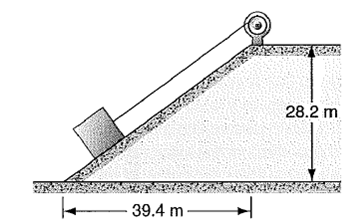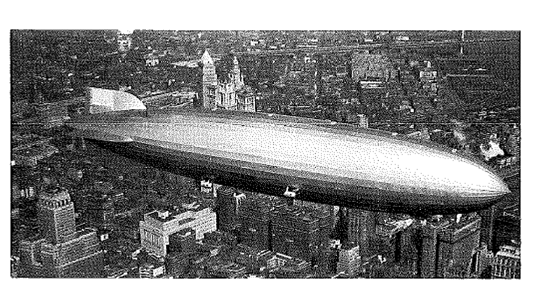×#### Thank you for registering.

One of our academic counsellors will contact you within 1 working day.

Click to Chat

1800-1023-196

+91-120-4616500

CART 0

• 0

MY CART (5)

Use Coupon: CART20 and get 20% off on all online Study Material

ITEM
DETAILS
MRP
DISCOUNT
FINAL PRICE
Total Price: Rs.

There are no items in this cart.
Continue Shopping• Complete JEE Main/Advanced Course and Test Series
• OFFERED PRICE: Rs. 15,900
• View Details

```Solved Examples on Work, Power & Energy

Question 1:- A 1380-kg block of granite is dragged up an incline at a constant speed of 1.34 m/s by a steam winch (below figure). The coefficient of kinetic friction between the block and the incline is 0.41. How much power must be supplied by the winch?Concept:

Weight of an object W is equal to the mass of the object times free fall acceleration g.

So, W=mg

The force of friction f is defined as,

f =µkN

Here µk is the coefficient of kinetic friction and N is the normal force.

Power P of an object is equal to the tension acting on the object T times velocity of the object v.  P=Tv

Solution:

The below figure shows a 1380-kg block of granite is dragged up an incline at a constant speed of 1.34 m/s by a steam winch.

Figure:From the figure,

tan θ = p/b

Or, θ = tan-1 (p/b)

To obtain angle θ, substitute 28.2 m for p and 39.4 m for b in the equation  θ = tan-1 (p/b),

θ = tan-1 (p/b)

= tan-1 (p/b)

= tan-1 (28.2 m/39.4 m)

= tan-1 (28.2 /39.4 )

=35.5°

The parallel component of the weight of the block   will be,

And the perpendicular component will be,

The force of friction on the block is,

f =µkN

To obtain the force of friction f on the block, substitute mgcos θ for N in the equation f =µkN,

f =µkN

= µk mgcos θ

So the tension T on the rope in order to move the block up the ramp will be,

To obtain the tension T on the rope, substitute mg sinθ for and µk mgcos θ for N in the equation ,

So the power P that must be supplied by the winch will be,

P = Tv

= mgv (sinθ +µk cosθ)

To obtain the power P that must be  supplied by the winch, substitute 1380 kg for m, 9.81 m/s2 for g, 1.34 m/s for v, 0.41 for µk and 35.5° for θ in the equation P = mgv (sinθ +µk cosθ),

P = mgv (sinθ +µk cosθ)

= (1380 kg) (9.81 m/s2) (1.34 m/s) [sin (35.5º) +(0.41) cos (35.5º)]

= (1.66×104 kg.m2/s3) [1 W/(1 kg.m2/s3)]

= 1.66×104 W

From the above observation we conclude that, the power P that must be  supplied by the winch would be  1.66×104 W.Question 2:-

The hydrogen-filled Hindenburg (see below figure) could cruise at 77 knots with the engines 4800 hp. Calculate the air drag force in newton’s on the airship at this speed.Concept:

Power delivered (P) by the body is equal to the force acting on the body (F) times speed of the body (v).

So, P = Fv…… (1)

From equation (1), the force (F) will be,

F = P/v   …… (2)

Solution:

To obtain air drag force in newtons, substitute 77 knots for v and 4800 hp for P in the equation F = P/v,

F = P/v

= 4800 hp/77 knots

= (4800 hp/77 knots) (745.7 W/1 hp) (1 knot/1.688 ft/s) (1 ft/s/0.3048 m/s)

= 9.0×104 W/m/s

= (9.0×104 W/m/s) [1 N/(1 W/m/s)]

=9.0×104 N   …… (3)

From the above observation we conclude that, air drag force in newtons on the airship at this speed will be 9.0×104 N.

Question 3:- A jet airplane is traveling 184 m/s. In each second the engine takes in 68.2 m3 of air having a mass of 70.2 kg. The air is used to burn 2.92 kg of fuel on each second. The energy is used to compress the products of combustion and to eject them at the rear of the engine at 497 m/s relative to the plane. Find (a) the thrust of the jet engine and (b) the delivered power (horse power) .

Concept:

Force or thrust F is equal to the rate of change of momentum (Δp/ Δt).

F = Δp/ Δt

Power P is defined as,

P = Fv

Here F is the force and v is the velocity.

Solution:

(a) First we have to find out the change in momentum Δp of the jet engine.

The change in momentum Δp will be,

Δp = (70.2 kg) (497 m/s-184 m/s) +(2.92 kg) (497 m/s

= 2.34×104kg.m/s

To obtain the thrust F of the jet engine, substitute 2.34×104kg.m/s for Δp and 1 s for Δt in the equation F = Δp/ Δt,

F = Δp/ Δt

= (2.34×104kg.m/s)/(1 s)

= (2.34×104kg.m/s2) (1 N/1 kg.m/s2)

=2.34×104 N

From the above observation we conclude that, the thrust F of the jet engine would be 2.34×104 N.

(b) To obtain the delivered power P of the jet engine, substitute 2.34×104 N for thrust F and 184 m/s for the velocity v of the jet airplane in the equation P = Fv, we get,

P = Fv

= (2.34×104 N) (184 m/s)

= (4.31×106N.m/s) (1 W/1 N.m/s)

= 4.31×106 W

= (4.31×106 W) (0.001341 hp/1 W)

= 5780 hp

From the above observation we conclude that, the delivered power P of the jet engine would be 5780 hp.

Question 4:- The string in below figure has a length L = 120 cm, and the distance d to the fixed peg is 75.0 cm. when the ball is released from rest in the position shown, it will follow the arc shown in the figure. How fast will it be going (a) when it reaches the lowest point in its swing and (b) when it reaches its highest point, after the string catches on the peg?Concept:

Kinetic energy K of an object is defined as,

K = ½ mv2

Here m is the mass of the object and v is the speed of the object.

Potential energy U is defined as,

U = mgy

Here, m is the mass of the object, g is the free fall acceleration and y is the height.

In accordance to conservation of energy, the total change in kinetic energy  ΔKtotal of all the particles that make up the system is equal in magnitude, but opposite in sign, to the total change in the potential energy -ΔUtotal of the system.

So, ΔKtotal= -ΔUtotal

K-K0= -(U-U0)

Solution:

Assume that, U0= K0 = 0

Substitute 0 for U0 and 0 for K0 in the equation K-K0= -(U-U0), we get,

K-0 = -(U-0)

So, K= -U

Substitute ½ mv2 for kinetic energy K and mgy for potential energy U in the equation

K= -U, we get,

K= -U

½ mv2= -mgy

v2/2 = -gy

Or, v = √2g(-y)

(a) To obtain the speed v of the ball, when it reaches the lowest point in its swing, substitute 9.81 m/s2 for free fall acceleration g and  -120 cm for y (negative sign for negative axis) in the equation v = √2g(-y), we get,

V = √2g(-y)

=√2(9.81 m/s2)(-(-120 cm))

= √2(9.81 m/s2)(120 cm)

=√2(9.81 m/s2)(120 cm)(10-2 m/1cm)

=4.85 m/s

From the above observation we conclude that, the speed v of the ball, when it reaches the lowest point in its swing would be 4.85 m/s.

(b) The value of y, when the ball reaches its highest point would be,

y = -[120 cm-(120 cm-75.0 cm)-(120 cm-75.0 cm)]

= -[120 cm- 45.0 cm- 45.0 cm]

= (- 30 cm) (10-2 m/1 cm)

= -0.30 m

To obtain the speed v of the ball, when the ball reaches its highest point, substitute 9.81 m/s2 for free fall acceleration g and  -0.30 m for y (negative sign for negative axis) in the equation v = √2g(-y), we get,

v = √2g(-y)

=√2(9.81 m/s2)(-(-0.30 m))

= √2(9.81 m/s2)(0.30 m)

=2.43 m/s

From the above observation we conclude that, the speed v of the ball, when the ball reaches its highest point, after the string catches on the peg would be 2.43 m/s.
```### Course Features

• 728 Video Lectures
• Revision Notes
• Previous Year Papers
• Mind Map
• Study Planner
• NCERT Solutions
• Discussion Forum
• Test paper with Video Solution